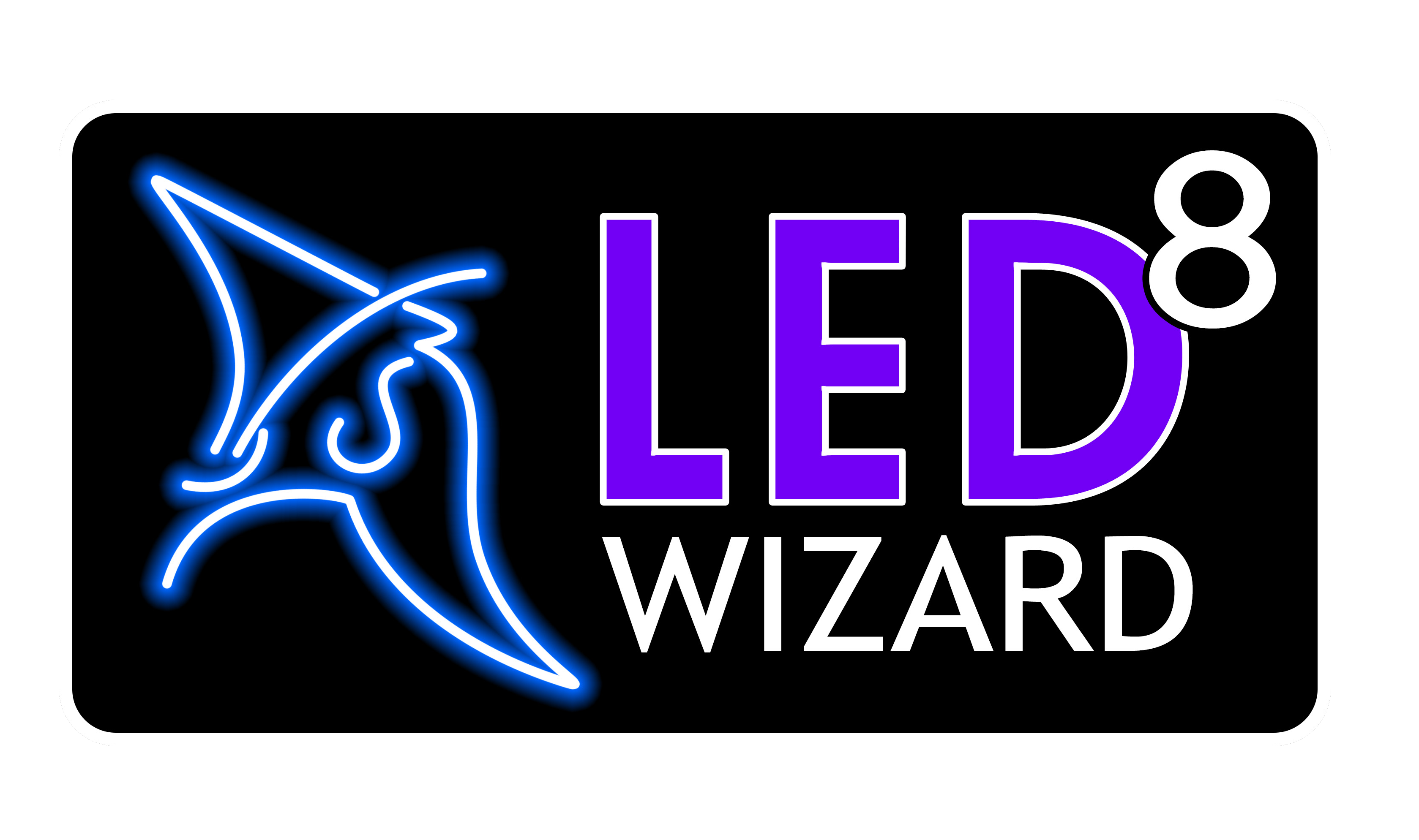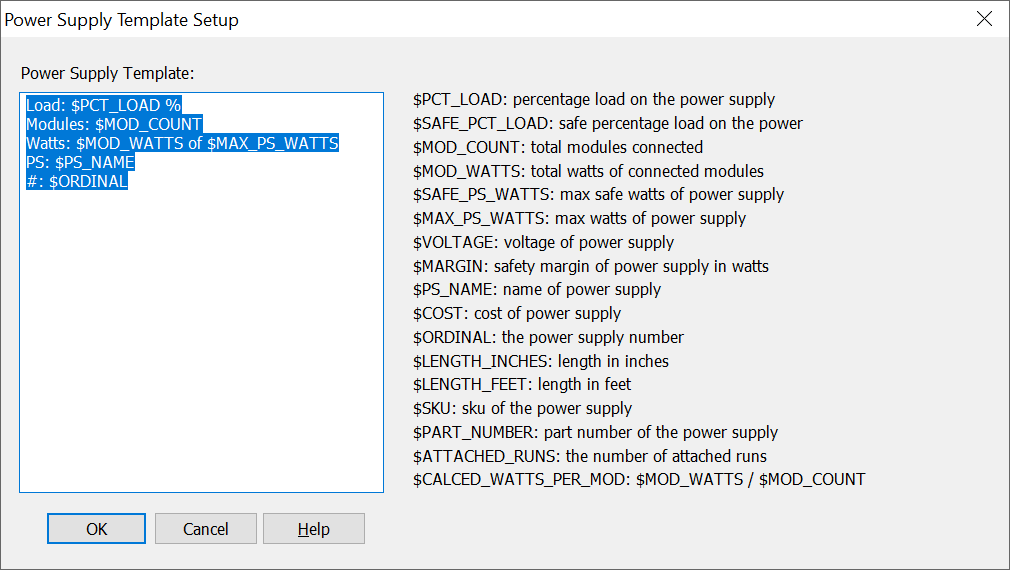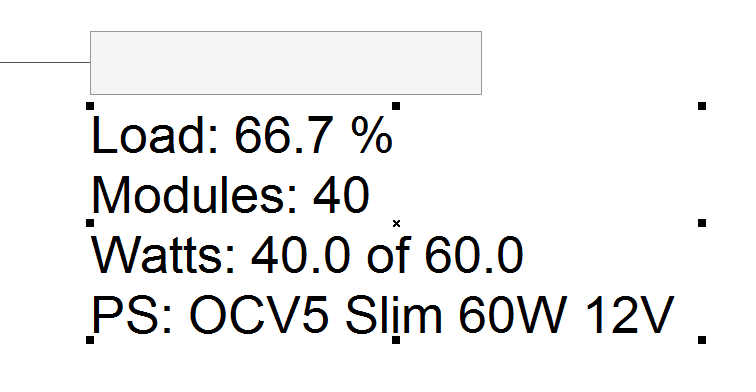LED Wizard 8 Documentation

## 8.15.25 Power Supply Template Setup

This dialog box lets you customize the power supply info text that appears beneath each power supply in your layouts.The large edit box on the left displays the template used when creating the power supply info text. You can rearrange the order and contents of each line as you wish.

The special variables that start with "\$" are replaced with the actual value. After the data variable name, you can add additional text labels, such as "%" in the first line for the load percentage.Please note that you can scale this power supply info by clicking on the text and then using the upper right control point. On larger layouts with many power supplies, sometimes this data can appear too small. The retangular box is the the actual size of the power supply and cannot be scaled.Below is a description of the variables:

This is the percentage load of the modules on the power supply, that is, the total watts divided by the total watts (not the safe watts) x 100.

This is the safe percentage load of the modules on the power supply, that is, the total watts divided by the total safe watts x 100.

##### \$LENGTH

This is the total length of the attached modules in a Flex layout.

##### \$MOD_COUNT

This is the total number of modules connected to the power supply.

##### \$MOD_WATTS

This is the total watts of all the modules connected to the power supply. It's simply the number of modules times the watts per module.

##### \$SAFE_PS_WATTS

This is the total safe watts of the power supply, which is the total watts minus the safety margin in watts.

##### \$MAX_PS_WATTS

This is the total watts of the power supply.

##### \$VOLTAGE

This is the voltage of this power supply, usually 12 or 24.

##### \$PS_MARGIN

This is the safety margin of this power supply in watts.

##### \$PS_NAME

This is the name of the power supply.

##### \$COST

This is the cost of this power supply.

##### \$ORGINAL

This is the power supply number assigned to it, that can appear in other parts of the title block.

##### \$LENGTH_INCHES

The length of the power supply in inches.

##### \$LENGTH_FEET

The length of the power supply in feet.

##### \$SKU

The power supply's SKU.

##### \$PART_NUMBER

The power supply's part number.

##### \$ATTACHED_RUNS

How many runs (rows) are attached to this power supply.

##### \$CALC_WATTS_PER_MOD

The total watts divided by the module count for this power supply.